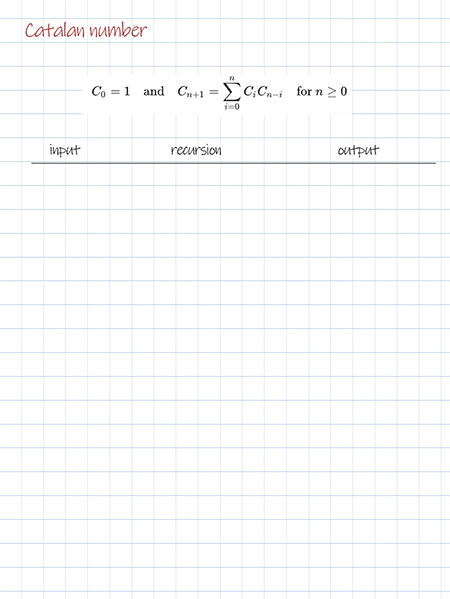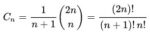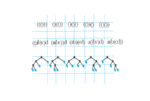In this post, we are going to find n-th Catalan number for any given n. There are 3 solutions. They are iteration, recursion and dynamic programming.

### Iterative solution

This solution is applying the following alternative expression of Cn. It can be implemented with iteration. It has the best time complexity O(n).

## Python

### Recursive solution

This solution is using the another alternative expression of Cn. It can be implemented with recursion. It has the worst time complexity O(2^n) with repeated calls.

## Doodle### Dynamic programming

This solution uses the same expression as recursion. But it overcomes the overlapping in recursion by using dynamic programming technique tabulation. The result from previous calls are saved to a 2d array. They can be re-used for the following steps without calling the recursion. It improves the time complexity from exponential to O(n^2).

## Python